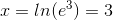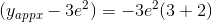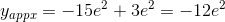# Calculus 1 : How to find prediction models

## Example Questions

### Example Question #1 : How To Find Prediction Models

Suppose you are a banker and set up a very unique function for your interest rate over time given by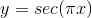However, you find your computer incapable of calculating the interest rate at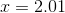. Estimate the value of the interest rate atby using a linear approximation, using the slope of the function at.UndefinedExplanation:

To do a linear approximation, we're going to create a function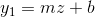, that approximates our situation. In our case, m will be the slope of the function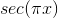at, while b will be the value of the functionat. The z will be distance from our starting position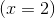to our end position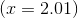, which is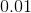Firstly, we need to find the derivative ofwith respect to x to determine slope.

By the power rule: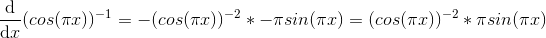The slope atwill therefore be 0 since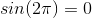.

Since this is the case, the approximate value of our interest rate will be identical to the value of the original function at x=2, which is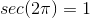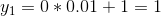### Example Question #2 : How To Find Prediction Models

Approximate the value at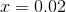of the function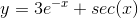,with a linear approximation using the slope of the function at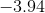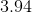Explanation:

To do this, we must determine the slope of the function at, which we will call, and the initial value of the function at, which we will call, and sinceis only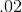away from, our linear approximation will look like: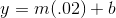To determine slope, we take the derivative of the function with respect to x and find its value at, which in our case is: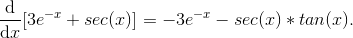At, our value forisTo determine, we need to determine the value of the original equation atAt, our value for b isSince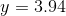### Example Question #3 : How To Find Prediction Models

Determine the tangent line to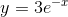at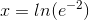, and use the tangent line to approximate the value at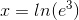.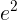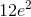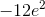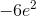Explanation:

First recall that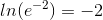To find the tangent line ofat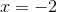, we first determine the slope of. To do so, we must find its derivative.

Recall that derivatives of exponential functions involving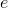are given as: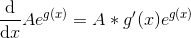, whereis a constant and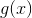is any function ofIn our case,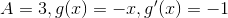,.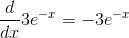At,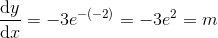, whereis the slope of the tangent line.

To use point-slope form, we need to know the value of the original function at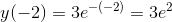Therefore,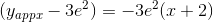At